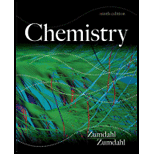# Given the values of ∆H and ∆ S , which of the following changes will be spontaneous at constant T and p ? a. ∆H = + 25 kJ, ∆S = + 5.0 J/K, T = 300. K b. ∆H = + 25 kJ, ∆ S = + 100. J/K, T = 300. K c. ∆H = − 10. kJ, ∆ S = + 5.0 J/K, T= 298 K d. ∆ H = − 10.kJ, ∆S =−40.J/K, T = 200.K### Chemistry

9th Edition
Steven S. Zumdahl
Publisher: Cengage Learning
ISBN: 9781133611097### Chemistry

9th Edition
Steven S. Zumdahl
Publisher: Cengage Learning
ISBN: 9781133611097

#### Solutions

Chapter
Section
Chapter 17, Problem 35E
Textbook Problem

## Expert Solution

### Want to see the full answer?

Check out a sample textbook solution.See solution

### Want to see this answer and more?

Experts are waiting 24/7 to provide step-by-step solutions in as fast as 30 minutes!*

See Solution

*Response times vary by subject and question complexity. Median response time is 34 minutes and may be longer for new subjects.